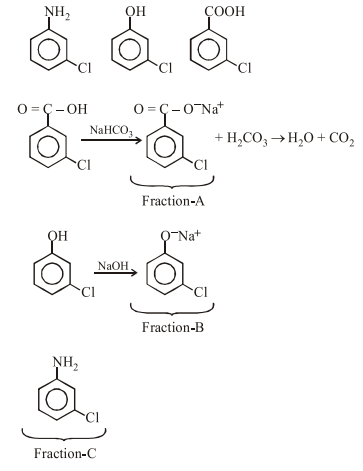# A solution of m-chloroaniline,

Question:

A solution of m-chloroaniline, $m$-chlorophenol and $\mathrm{m}$-chlorobenzoic acid in ethyl acetate was extracted initially with a saturated solution of $\mathrm{NaHCO}_{3}$ to give fraction A. The left over organic phase was extracted with dilute $\mathrm{NaOH}$ solution to give fraction B. The final organic layer was labelled as fraction C. Fractions A, B and C, contain respectively :

1. m-chlorobenzoic acid, m-chloroaniline and $\mathrm{m}$-chlorophenol

2. m-chloroaniline, m-chlorobenzoic acid and $\mathrm{m}$-chlorophenol

3. m-chlorobenzoic acid, m-chlorophenol and $\mathrm{m}$-chloroaniline

4. m-chlorophenol, m-chlorobenzoic acid and m-chloroaniline

Correct Option: , 3

Solution: